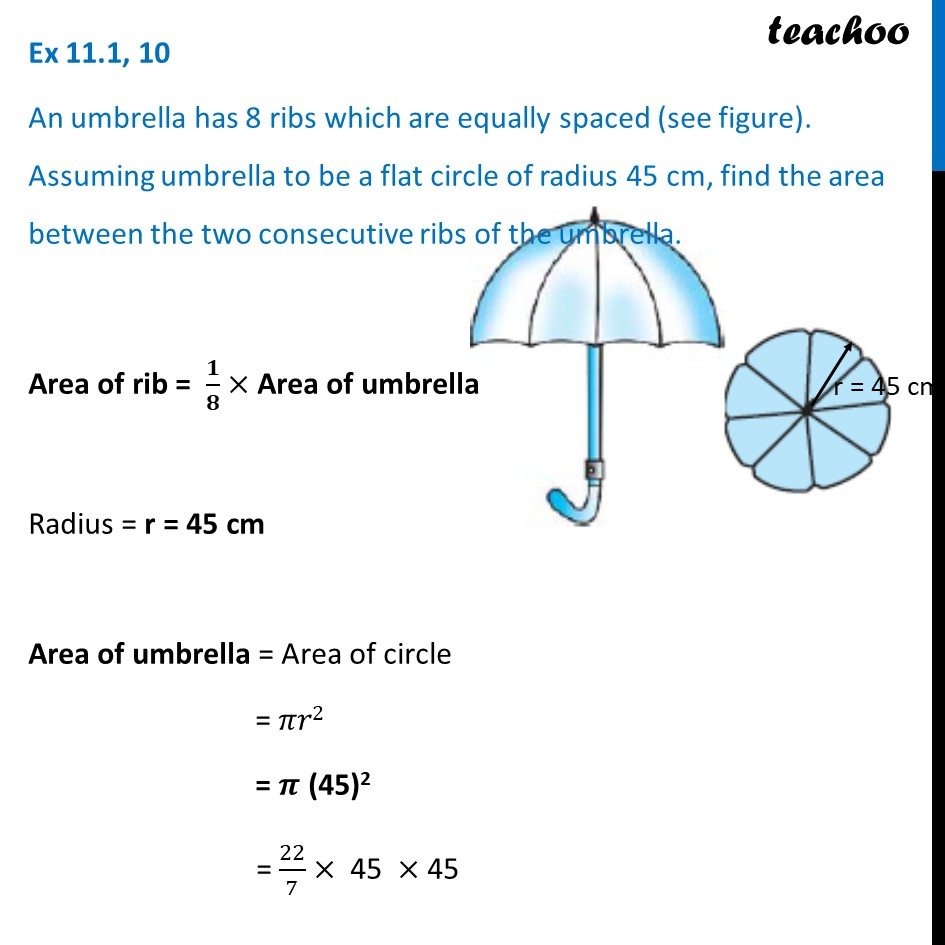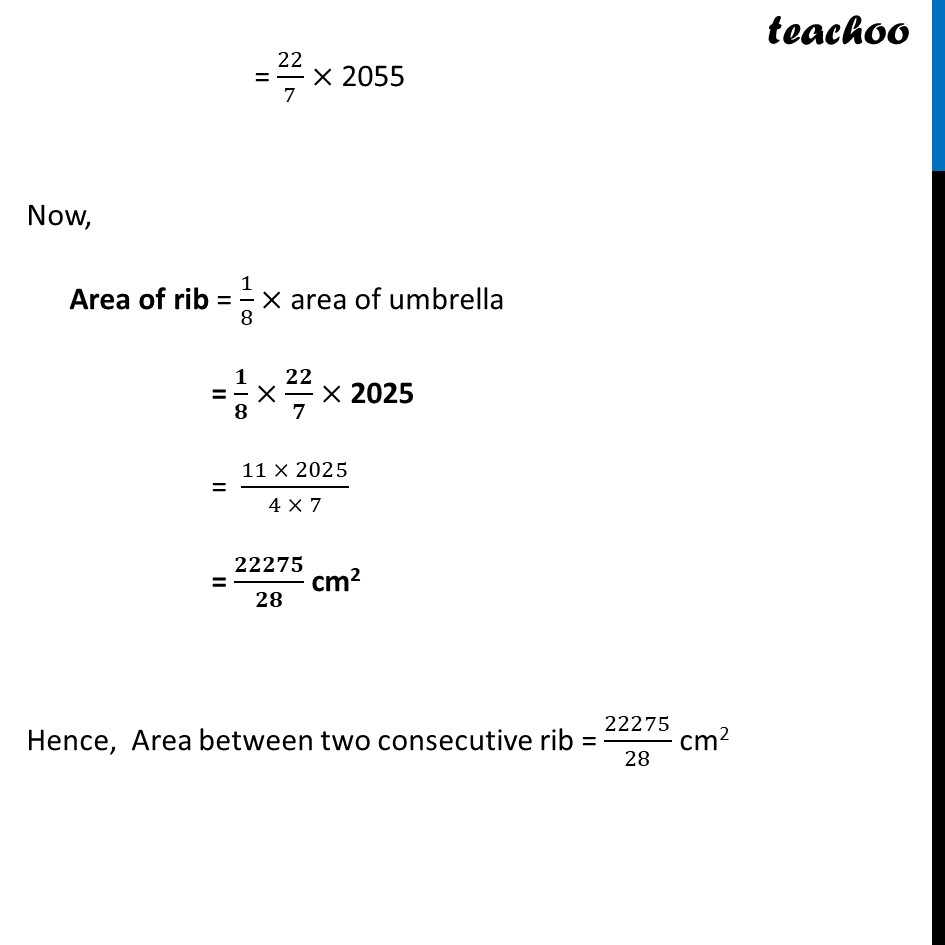Area of sector of circle

Chapter 11 Class 10 Areas related to Circles
Concept wiseLearn in your speed, with individual attention - Teachoo Maths 1-on-1 Class

### Transcript

Ex 11.1, 10 An umbrella has 8 ribs which are equally spaced (see figure). Assuming umbrella to be a flat circle of radius 45 cm, find the area between the two consecutive ribs of the umbrella. Area of rib = 𝟏/𝟖× Area of umbrella Radius = r = 45 cm Area of umbrella = Area of circle = 𝜋𝑟2 = 𝝅 (45)2 = 22/7× "45 "× 45 = 22/7× 2055 Now, Area of rib = 1/8× area of umbrella = 𝟏/𝟖×𝟐𝟐/𝟕× 2025 = (11 × 2025)/(4 × 7) = 𝟐𝟐𝟐𝟕𝟓/𝟐𝟖 cm2 Hence, Area between two consecutive rib = 22275/28 cm2